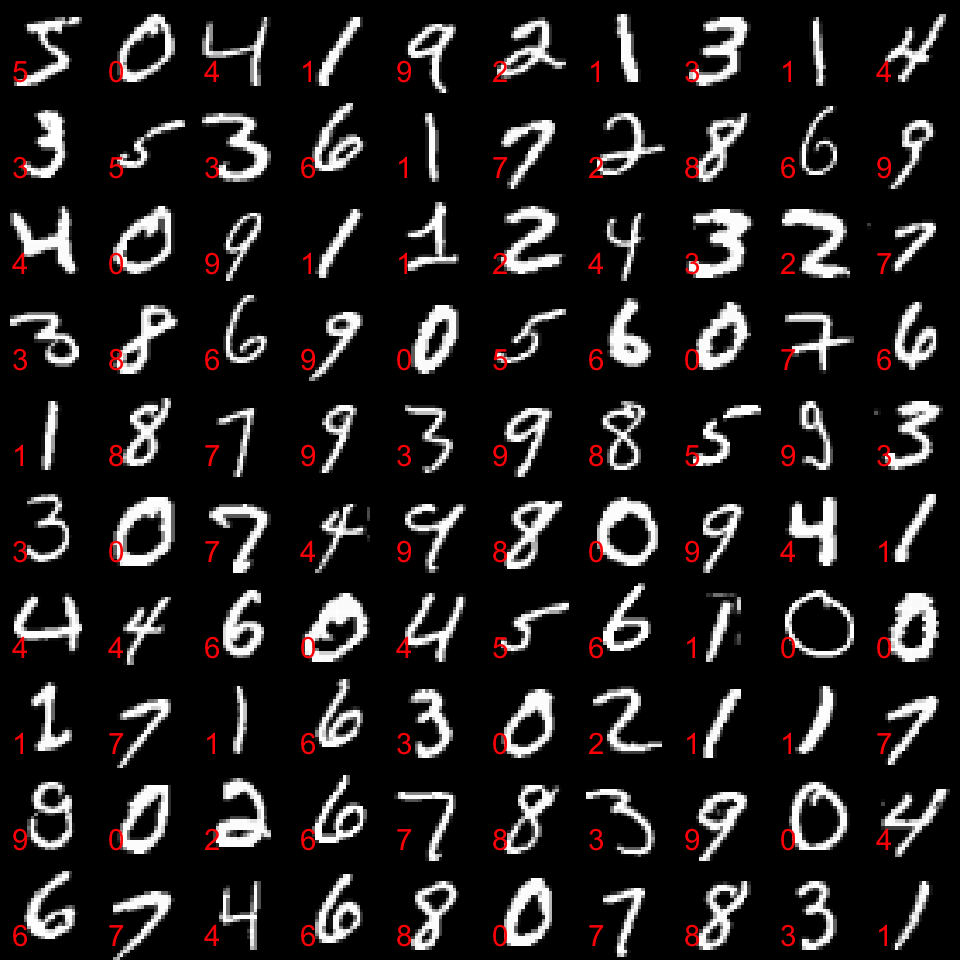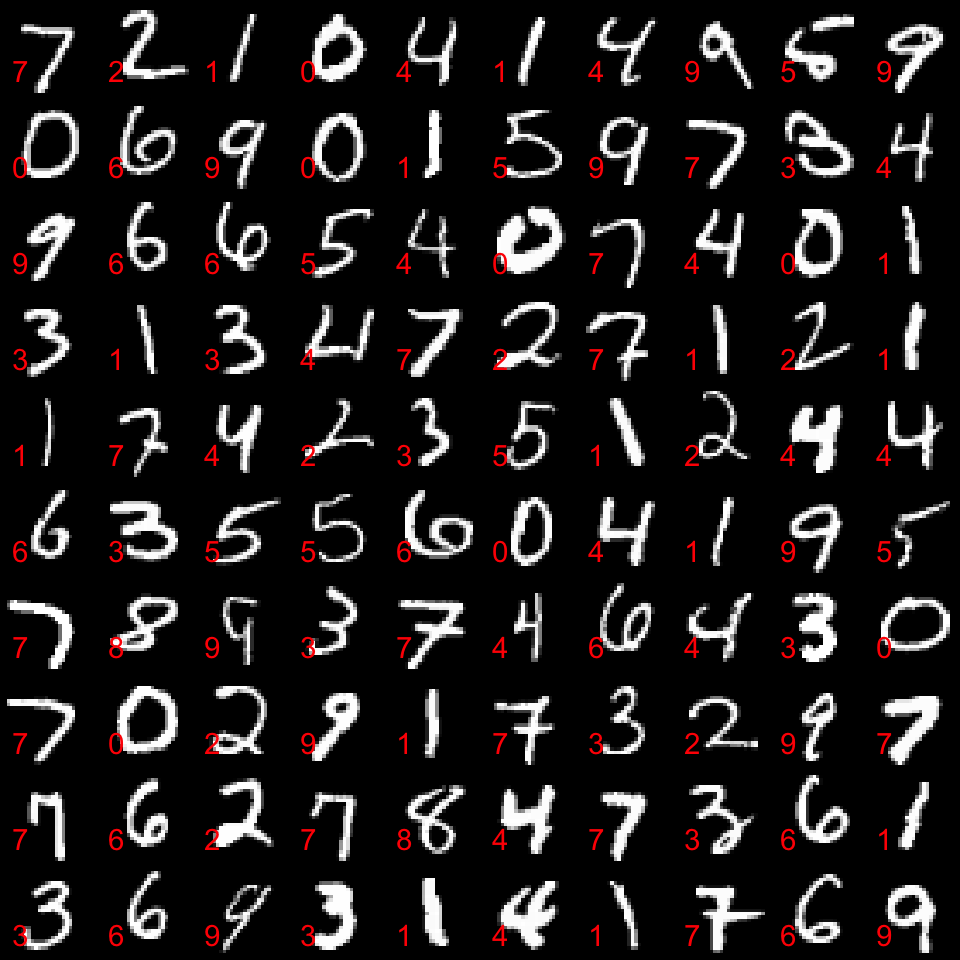## Introduction

This document (R-Markdown file) is made for the R-part of the MathematicaVsR project “Handwritten digits classification by matrix factorization”.

The main goal of this document is to demonstrate how to do in R: - the ingestion images from binary files the MNIST database of images of handwritten digits, and - using matrix factorization to built a classifier, and - classifier evaluation by accuracy and F-score calculation.

The matrix factorization methods used are Singular Value Decomposition (SVD) and Non-negative Matrix Factorization (NMF).

## Concrete steps

The concrete steps taken follow.

1. Ingest the binary data files into arrays that can be visualized as digit images.
• The MNIST database have two sets: $$60,000$$ training images and $$10,000$$ testing images.
1. Make a linear vector space representation of the images by simple unfolding.

2. For each digit find the corresponding representation matrix and factorize it.

3. Store the matrix factorization results in a suitable data structure. (These results comprise the classifier training.)

• One of the matrix factors is seen as a new basis.
1. For a given test image (and its linear vector space representation) find the basis that approximates it best. The corresponding digit is the classifier prediction for the given test image.

2. Evaluate the classifier(s) over all test images and compute accuracy, F-Scores, and other measures.

More details about the classification algorithm are given in the blog post “Classification of handwritten digits”, . The method and algorithm are described Chapter 10 of .

## Libraries and source code used

library(plyr)
library(ggplot2)
library(irlba)
library(MASS)
library(data.table)
library(doParallel)
library(devtools)
source_url("https://raw.githubusercontent.com/antononcube/MathematicaForPrediction/master/R/NonNegativeMatrixFactorization.R")
## SHA-1 hash of file is e8ee968e4c02573c76a248e9835bd151c80a5cce

## Details of the classification algorithm

### Training phase

1. Optionally re-size, blur, or transform in other ways each training image into an array.
2. Each image array (raster image) is linearized — the rows (or columns) are aligned into a one dimensional array. In other words, each raster image is mapped into a $$\mathbf{R}^m$$ vector space, where $$m$$ is the number of pixels of a transformed image.
• We will call these one dimensional arrays image vectors.
1. From each set of images corresponding to a digit make a matrix with $$m$$ columns of the corresponding image vectors.
2. Using the matrices in step 3 use a thin Singular Value Decomposition (SVD) to derive orthogonal bases that describe the image data for each digit.

### Classification phase

1. Given an image of an unknown digit derive its image vector $$v$$ in the same was as in the training phase.
2. Find the residuals of the approximations of $$v$$ with each of the bases found in step 4 of the training phase.
3. The digit with the minimal residual is the classification result.

### Using Non-negative Matrix Factorization

In order to use Non-negative Matrix Factorization (NMF) instead of SVD, the classification phase has to be modified since the obtained bases are not orthogonal. See below for theoretical and algorithmic details.

## Handwritten digits data ingestion

First we download the files given in the MNIST database site :

### Definitions of integestion functions

The following code follows very closely the R code for MNIST ingestion written by Brendan O’Connor (brendano).

ReadMNISTImages <- function( fileName, .progress = "none" ) {

## magic number, number of images, number of rows, number of columns
names(readInfo) <- c("MNum", "NImages", "NRows", "NColumns")

images <-
llply( 1:readInfo$NImages, function(i) { mat <- matrix( readBin( toRead, 'integer', size = 1, n= readInfo$NRows * readInfo$NColumns, endian="big", signed=F ), readInfo$NRows, readInfo$NColumns ) mat[, nrow(mat):1] }, .progress = .progress ) close(toRead) images } ReadMNISTImageLabels <- function( fileName ) { toRead <- file(fileName, "rb") readLabelsInfo <- as.list( readBin( toRead, 'integer', n=2, size=4, endian="big") ) names(readLabelsInfo) <- c("MNum", "NImages") labels = readBin(toRead, 'integer', n = readLabelsInfo$NImages, size=1, signed=F )

labels
}

### Ingestion

if( FALSE || !exists("trainImages") ) {

cat( "\tTime to read training images :", system.time( {
} ), "\n")

cat( "\tTime to read test images :", system.time( {
} ), "\n")

names(trainImages) <- paste("train", 1:length(trainImages), sep = "-" )
names(trainImagesLabels) <- paste("train", 1:length(trainImages), sep = "-" )

names(testImages) <- paste("test", 1:length(testImages), sep = "-" )
names(testImagesLabels) <- paste("test", 1:length(testImages), sep = "-" )
}

### Verification statistics and plots

#### Training set

Number of images per digit for the training set:

count(trainImagesLabels)
##    x freq
## 1  0 5923
## 2  1 6742
## 3  2 5958
## 4  3 6131
## 5  4 5842
## 6  5 5421
## 7  6 5918
## 8  7 6265
## 9  8 5851
## 10 9 5949

Visualize the first $$100$$ images with their labels of the training set:

par( mfrow = c(10,10), mai = c(0,0,0,0))
for(i in 1:100){
image( trainImages[[i]], axes = FALSE, col = gray( 0:255 / 255 ) )
text( 0.2, 0, trainImagesLabels[[i]], cex = 1.4, col = 2, pos = c(3,4))
}(If order to make the plot above with inverted colors use ‘col = gray( 255:0 / 255 )’.)

#### Testing set

Number of images per digit for the testing set:

count(testImagesLabels)
##    x freq
## 1  0  980
## 2  1 1135
## 3  2 1032
## 4  3 1010
## 5  4  982
## 6  5  892
## 7  6  958
## 8  7 1028
## 9  8  974
## 10 9 1009

Visualize the first $$100$$ images with their labels of the testing set:

par( mfrow = c(10,10), mai = c(0,0,0,0))
for(i in 1:100){
image( testImages[[i]], axes = FALSE, col = gray( 0:255 / 255 ) )
text( 0.2, 0, testImagesLabels[[i]], cex = 1.4, col = 2, pos = c(3,4))
}(If order to make the plot above with inverted colors use col = gray( 255:0 / 255 ).)

## Linear vector space representation

Each image is flattened and it is placed as a row in a matrix.